Scientific Communication As Sequential Art

Bret Victor / May 24, 2011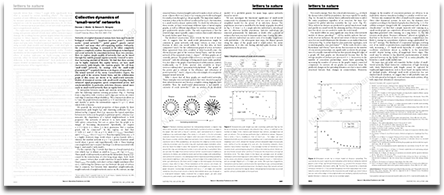This page presents a scientific paper that has been redesigned as a sequence of illustrations with captions. This comic-like format, with tightly-coupled pictures and prose, allows the author to depict and describe simultaneously — show and tell.

It is based on Watts and Strogatz's seminal Nature paper on network theory, shown to the right. This paper was chosen because it's accessible to a broad audience, and it's very well-written — already near the limit of clarity for just prose.

Try actually reading this design instead of skimming it (it's quicker than it looks!) and consider how the illustrations make abstract descriptions more concrete, and help you maintain the "picture in your head".

Further discussion follows.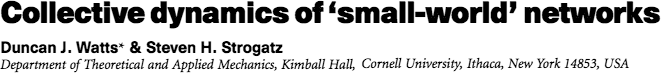ABSTRACT

Networks of coupled dynamical systems have been used to model biological oscillators, Josephson junction arrays, excitable media, neural networks, spatial games, genetic control networks and many other self-organizing systems. Ordinarily, the connection topology is assumed to be either completely regular or completely random. But many biological, technological and social networks lie somewhere between these two extremes.

Here we explore simple models of networks that can be tuned through this middle ground: regular networks 'rewired' to introduce increasing amounts of disorder. We find that these systems can be highly clustered, like regular lattices, yet have small characteristic path lengths, like random graphs. We call them 'small-world' networks, by analogy with the small-world phenomenon (popularly known as six degrees of separation). The neural network of the worm Caenorhabditis elegans, the power grid of the western United States, and the collaboration graph of film actors are shown to be small-world networks.

Models of dynamical systems with small-world coupling display enhanced signal-propagation speed, computational power, and synchronizability. In particular, infectious diseases spread more easily in small-world networks than in regular lattices.

ALGORITHM

To interpolate between regular and random networks, we consider the following random rewiring procedure.

ring of n vertices

n = 12

where each vertex
is connected to its
k nearest neighbors

k = 4

like so.

We choose a vertex, and
the edge to its nearest
clockwise neighbour.

With probability p, we reconnect
this edge to a vertex chosen
uniformly at random over the

entire ring, with
duplicate edges
forbidden. Other-
wise, we leave
the edge in place.

We repeat this process by
moving clockwise around
the ring, considering each

vertex in turn
until one lap
is completed.

Next, we consider the
edges that connect vertices
to their second-nearest
neighbours clockwise.

As before, we randomly
rewire each of these
edges with probability p.

We continue this process,
circulating around the ring and
proceeding outward to more
distant neighbours after each
lap, until each original edge
has been considered once.

As there are nk/2 edges in
the entire graph, the rewiring
process stops after k/2 laps.

For p = 0,
the ring is
unchanged.

As p increases, the
graph becomes
increasingly disordered.

p=0.01

At p = 1, all
edges are re-
wired randomly.

This construction allows us to 'tune' the graph between regularity (p = 0) and disorder (p = 1), and thereby to probe the intermediate region 0 < p < 1, about which little is known.

METRICS

We quantify the structural properties of these graphs by their characteristic path length L(p) and clustering coefficient C(p).
L(p) measures the typical separation between two vertices (a global property). C(p) measures the cliquishness of a typical neighbourhood (a local property).

L is defined as the number
of edges in the shortest
path between two vertices

shortest path
is 1 edge

shortest path
is 3 edges

averaged over all
pairs of vertices.

C is defined as follows.
Suppose that a vertex v
has kv neighbours.

kv = 4 neighbours

Then at most kv (kv – 1) / 2 edges
can exist between them. (This
occurs when every neighbor of

v is connected
to every other
neighbour of v.)

at most 6 edges between 4 neighbours

Let Cv denote the fraction of
these allowable edges that
actually exist. Define C as the

average of Cv
over all vertices.

4 out of 6 edges exist. Cv = 4/6 = 0.67

For friendship networks, these statistics have intuitive meanings: L is the average number of friendships in the shortest chain connecting two people.
Cv reflects the extent to which friends of v are also friends of each other; and thus C measures the cliquishness of a typical friendship circle.

SMALL
WORLDS

The regular lattice at p = 0 is
a highly clustered, large world
where L grows linearly with n.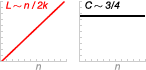The random network at p = 1 is a
poorly clustered, small world where
L grows only logarithmically with n.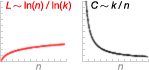These limiting cases might lead one to suspect that large C is always associated with
large L, and small C with small L. On the contrary, we find that there is a broad
interval of p over which L(p) is almost as small as Lrandom yet Cp >> Crandom.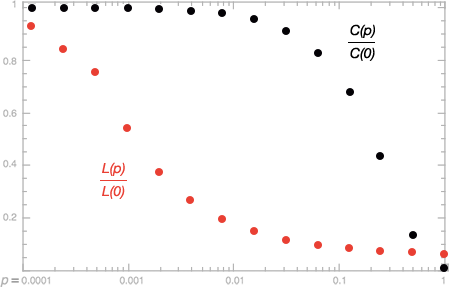The data shown in the figure are averages over 20 random realizations of the rewiring process, and have been normalized by the values L(0), C(0) for a regular lattice. All the graphs have n = 1000 vertices and an average degree of k = 10 edges per vertex. We note that a logarithmic horizontal scale has been used to resolve the rapid drop in L(p), corresponding to the onset of the small-world phenomenon. During this drop, C(p) remains almost constant at its value for the regular lattice, indicating that the transition to a small world is almost undetectable at the local level.

These small-world networks result from the immediate drop
in L(p) caused by the introduction of a few long-range edges.
Such 'short cuts' connect vertices that would otherwise be
much farther apart than Lrandom. For small p, each short

cut has a highly nonlinear
effect on L, contracting the
distance not just between the
pair of vertices that it
connects, but between their
immediate neighbourhoods,
neighbourhoods of neigh-
bourhoods and so on.

5 hops to
neighbourhood

shortcut to
neighbourhood

By contrast, an edge removed from a clustered neighbour-
hood to make a short cut has, at most, a linear effect on C;
hence C(p) remains practically unchanged for small p even
though L(p) drops rapidly. The important implication here is

that at the local level (as
reflected by C(p)), the trans-
ition to a small world is
almost undetectable.

The 4 neighbors of
each vertex have
3 out of 6 edges
among themselves.
C = 3/6 = 0.5

With shortcut,
this is still true
for almost
every vertex.
C = 0.48

(The paper goes on to identify several real-life networks as small-world, and discuss the implications for dynamical systems. I apologize for omitting the good stuff.)

Some notes:

A computer screen is not space-constrained like a physical journal. We can use as many pixels as we need to explain a concept. Paradoxically, making lavish use of space can result in briefer writing — illustrations allow for terser prose. The word count (and reading time) of a graphic novel is far less than that of a comparable prose novel.

When an algorithm is described in prose (or code), we are typically given only the rules of the system — we can't see the data or the state. In order to understand what the algorithm is doing, we have to "play computer" and imagine the state in our head. Illustrating the state of an algorithm at each step can make the description dramatically easier to follow.

I've used a small amount of lightweight interactivity (via hover-sliders) to allow the reader to see the progression of the algorithm, in-place, over many steps. This interaction is optional and supplemental — it's available for a reader who wants the clarification, but can be skipped by a reader who already understands (or doesn't care). For more on subtle supplemental interactivity, see Explorable Explanations.

The roles of pictures and prose can be fluid. For the description of the algorithm, pictures are coupled to individual sentences or even fragments, and the words serve to explain the action in the pictures: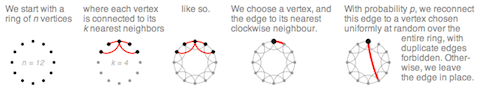Whereas, for the material near the end, pictures are coupled to entire paragraphs, and the pictures serve as examples for concepts conveyed by words: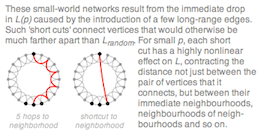Finally, "comic-style" needn't mean characters, dialog, word balloons, or sound effects. Let alone superheroes or anthropomorphic animals. The comic form is about sequences of tightly-integrated words and pictures, together conveying a message more powerfully than the sum of their parts. The potential of this form to explain difficult concepts is unmatched and underused.

For another comic-style explanation (this time, an art analysis), see Tom Oreb's Portrait of Ward Kimball.